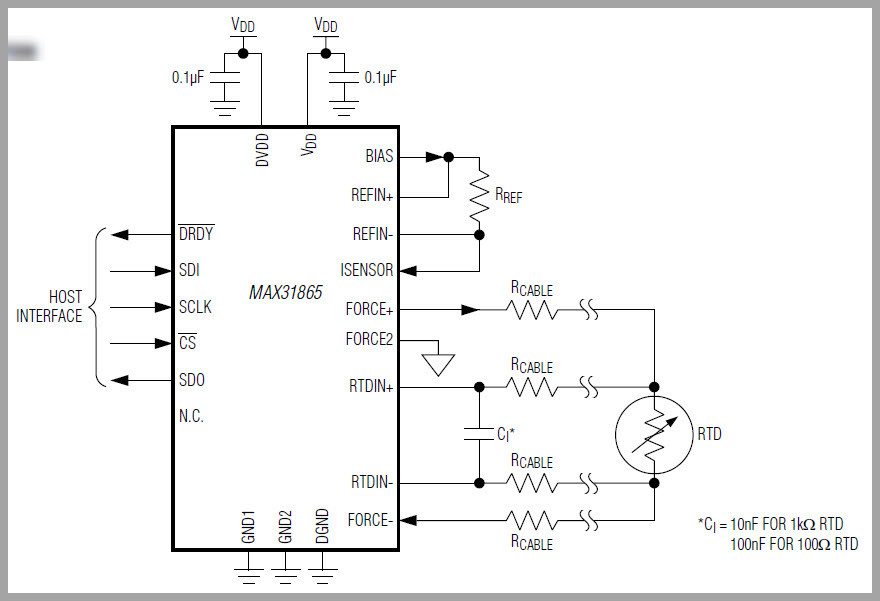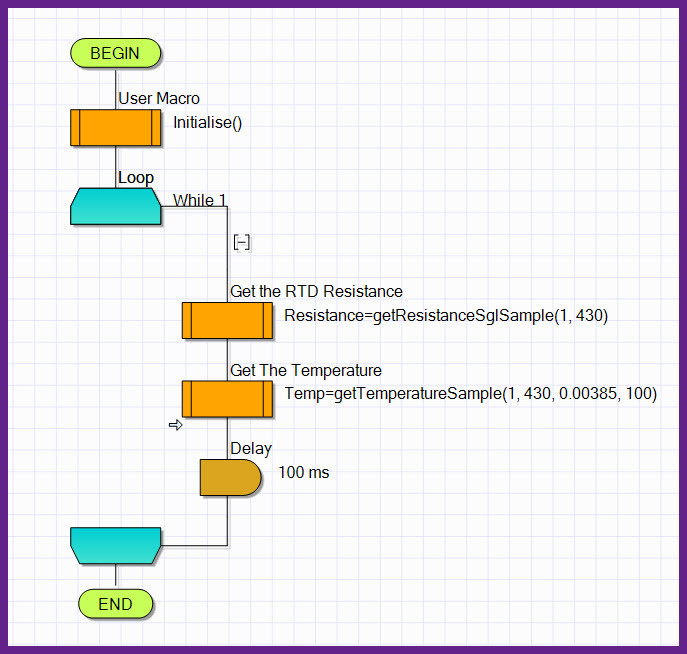Search

# Temperature Measurements with RTD Sensors (MAX31865)

In this short article we will take a look at the MAX31865 Resistance to Temperature Converter and it's use in temperature applications, we also look at RTD basics and their use in use Flowcode.

The MAX31865 is an easy-to-use resistance-to-digital converter optimized for platinum resistance temperature detectors (RTDs). An external resistor sets the sensitivity for the RTD being used and a precision delta-sigma ADC converts the ratio of the RTD resistance to the reference resistance into digital form. The MAX31865’s inputs are protected against overvoltage faults as large as 45V. Programmable detection of RTD and cable open and short conditions is included. Suitable for accurately measuring Hight temperatures such Turbo Exhaust Gas temperatures as shown below with Temperature up to around 1000°CTurbocharger on Testbed 970°C

Simple Conversion of Platinum RTD Resistance to Digital Value, Handles 100Ω to 1kΩ (at 0°C) Platinum RTDs (PT100 / PT1000)

Compatible with 2-, 3-, and 4-Wire Sensor Connections

SPI-Compatible Interface

High Accuracy Facilitates Meeting Error Budgets

15-Bit ADC Resolution; Nominal Temperature Resolution 0.03125NC (Varies Due to RTD Nonlinearity).Total Accuracy Over All Operating Conditions: 0.5NC (0.05% of Full Scale) max

Fully Differential VREF Inputs,21ms (max) Conversion Time.

Integrated Fault Detection Increases System Reliability, ±45V Input Protection.

Fault Detection (Open RTD Element, RTD Shorted to Out-of-Range Voltage, or Short Across RTD Element)

Communication Interface - SPI.### RTD Sensors

RTDs are really very simple devices: just a small strip of Platinum that measures 100Ω or 1000Ω exactly at 0°C. Bonded to the PT100/PT1000 are 2, 3 or 4 wires. called 2-Wire,3-Wire or 4-Wire RTD. We look at the 3 wire a bit closer however it is the same for the different RTD's. They come in 2 flavors PT100 and PT1000

Compared to most NTC/PTC thermistors, the PT type of RTD is much more stable and precise ( also more expensive) PT's have been used for many years to measure temperature in laboratory and industrial processes, and have developed a reputation for accuracy (better than thermocouples), repeatability, and stability. To get that precision and accuracy out of the PT100x RTD an amplifier that is designed to read the low resistance must be used. Even better to have an amplifier that can automatically adjust and compensate for the resistance of the connecting wires. This is the MAX31865 sensor.

The MAX31865 handles all of our RTD needs, and can compensate 3 or 4 wire RTDs for better accuracy. Connect to it with any microcontroller over SPI and read out the resistance ratio from the internal ADC and calculate the corresponding temperature. We are using Flowcode as our Programming Language to do the communication to the MAX32865 and calculate the temperature.

### PT100 RTD

A PT100 normally has 3 wires. It is in simple terms a resistance that changes with temperature. It is called a PT100 because at 0°C it will measure 100 ohms. At ambient it will be around 138 ohms.(PT1000 = 1000Ohm)

Because a very small change in resistance happens with each degree in temperature the added resistance of the wires will cause an error when connecting to a temperature controller. In order to eliminate the effect of the wires ,we need at least 3 wires.

The controller will measure the wire resistance and subtract it to leave us with just the PT100 resistance.

In our sketch below we have the following resistances, Rpt, R1, R2 and R3. Mathematically the controller does the following calculation. Rpt = “the measurement between A and B” less “the resistance measured between B and C” 10. So Rpt = (R1 + Rpt + R2) – (R2 + R3)it is important where each wire is connected. Normally the wires will be coloured so that 2 wires are the same colour and a third is different. Wire A could be white and wire B and wire C may be another colour say Red.

To determine wire connection use a multi-meter and measure the Resistance measured between the wires between one set of 2 Wires you should read the Resistance approx. 100 Ohm and between the another two wires low resistance (almost short circuit)which is the loop or compensation wire B-B. as seen in the Next Picture.PT100 Sensor

### Connecting the PT100 to the MAX31865 and Board setup

We used the Adafruit breakout board for the MAX31865 and a PT100,it should be connected to the board and configured as follows.

Connect the three wires to the three right-most contacts. Use a multimeter to determine which wires connect together directly (<2 ohms or so between them) and which connect through the RTD. Chances are the wires that connect together are the same color. The two wires that are connected together should go in the right-most blocks (F+ and RTD+). It doesn't matter which of the matched pair is on the outside or inside. The third wire that is on the other side of the RTD connects to the left (F- or RTD-). It doesn't matter which slot it's in!You will have to cut the thin trace in between the 2-way jumper on the right side of the board, and then solder closed the blob on the right side.

Then next to the terminal block on the left, solder closed that jumper as well. Alternatively you can put a piece of wire into the terminal blocks to 'short' them

There is a 0.1% resistor as a reference resistor on the breakout.

• The PT100 version of the breakout uses 430Ω

• The PT1000 version uses 4300Ω

Detailed description of the board can be found here

### Power Pins:

• Vin - this is the power pin. Since the chip uses 3 VDC, there is a Voltage regulator on the board you can connect 3 or 5V to Vin.

• 3Vo - this is the 3.3V output from the voltage regulator,

• GND - common ground for power and logic

### Hardware SPI Logic pins:

All pins going into the breakout board have level shifting circuitry to make them 3-5V logic level safe. Use whatever logic level is on Vin!

• SCK - This is the SPI Clock pin, its an input to the chip

• SDO - this is the Serial Data Out / Microcontroller In Sensor Out pin, for data sent from the MAX31865 to the microcontroller

• SDI - this is the Serial Data In / Microcontroller Out Sensor In pin, for data sent from the microcontroller to the MAX31865

• CS - this is the Chip Select pin, pull it low to start an SPI transaction. Its an input to the microcontroller.

### Using an Arduino board Hardware SPI, Connections is as follows

• Connect Vin to the power supply, 3V or 5V, Use the same voltage that the microcontroller logic is based on. For most Arduino's, that is 5V

• Connect GND to common power/data ground

• Connect the CLK pin to PIN 13

• Connect the SDO(MISO) pin to PIN 12

• Connect the SDI(MOSI) pin to PIN 11

• Connect the CS pin to PIN 10 (any pin can be used)

• Connect the RDY pin PIN 8(any pin can be used)SPI Connections

Other Micro-controller users should look at the Flowcode property settings for the corresponding pins the respective micro is using.

### Flowcode.

There is a Software Component available for Flowcode to work and use the MAX31865 Resistance to Temperature Converter, in the shop of FlowcodeXchange.com that makes it easy to read Resistance and Temperatures from the MAX31865.

We take a a look at the component how to set it up and use the component in the next few paragraphs.

The component uses an algorithm to calculate temperature. which works very well. However there are advanced methods which could improve accuracy which users can search for and implement in Flowcode if they wish to do so.

### Calculating the Resistance from the Ratio

The MAX31865 returns the Ratio of Resistance(reference vs RTD Resistance ) in a unsigned 16-bit number 0XFFFF which is Ratio 1.0 it is easy to get the resistance Ratio

Number returned from The MAX/ 32768 = Ratio

Example MAX31865 send 8292/ 32768 = 0.25305

If you are using a PT100 the Resistance Reference is 430 Ohm

then the Resistance of the RTD is given by

Resistance (RTD) = R_REF * Ratio

430 * 0.25305

108.811 Ohm

### Calculating the the Temperature with the RTD Measured Temperature

Resistive Temperature Detectors (RTDs) relate resistance to temperature by the following formula: RT = Rref[1 + α(T − Tref)] Where, RT = Resistance of RTD at given temperature T (ohms) Rref = Resistance of RTD at the reference temperature Tref (ohms)

The two most widely used values for alpha(α) are 0.00385 and 0.00392, corresponding to the IEC 751 (PT100) and SAMA standards.The Datasheet of your RTD Sensor should/will specify this alpha value (α)RTD Curve Resistance Vs Temperature

Note :There are many ways to implement and get the Temperature from the RTD resistance such as using lookup tables and using polynomial calculation however the method used in the Flowcode component has a relatively high accuracy and should serve purpose for many applications,The source code is included for Advanced users that would like to improve on the Accuracy. As we can see from the above graph RTD curves are not 100% linear and that the reason that Lookup tables and Polynomial can improve curacy

The Componet supports Two macro Calls.

• getTemperatureSample() This macro Calculates the Temperature and return the Temperature value.The user has to specify the parameters for the RTD in use

1. RTD Type 2/4 or 3 - Wire

2. The Resistance of the Reference Resistor Used

3. Temperature Coefficient for the RTD (Normally as per IEC 751 = 0,00385)

4. RTD_REF Temperature for PT100 this 100 at 0°C

5. Returned variable to save the Temperature Value• getResistanceSglSample() This macro Returns the Resistance of the RTD as above the user has to enter the following Parameters

1. RTD_Type 2/4 or 3-Wire RTD

2. R_REF the Resistance value of the Reference Resistor

3. Returned Variable for the Resistance Value of the RTD• Reading the Error Status Register

The User can call the macro getErrorStatus() which returns a a byte with bit fields

The user need to mask out the various bits if errors is present to determine which

Error is present. This Bits and there Error representation is Shown in the device datasheet .• Threshold Register Settings

The Threshold Registers are set at the Default settings as per Datasheet High Threshold = 0xFFFF and the Low Threshold = 0x0000,If the User application needs

these advanced settings for error detection it can be included by the User.

These settings cover most general applications

### Using the Macro's in a Flowcode Chart.Flowcode Example

### Flowcode Simulation

The Component does not support Panel Simulation however it supports SCADA Simulation

by using an SCADA device and a SPI Injector Component you can communicate with the MAX31865 and have the data in your flowcode simulation as shown below.

See our Blog on SCADA Simulation in Flowcode.### Simulation Results in FC Watch Window .### Note: The Source Coce were tested in SCADA Simulation

• UNO R3

• PT100 RTD Sensor

• SPI Injector in FC

• SCADA Slave Component in FC.